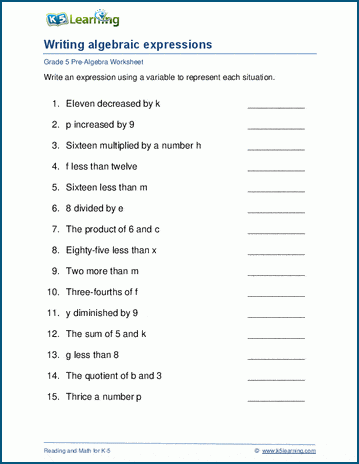## Introducing algebra

Our grade 5 pre-algebra worksheets introduce the use of variables in expressions and equations. Worksheets include one and two variable expressions, simplifying expressions and solving equations.

 Algebra vocabulary Expressions with one variable x + 12 Expressions with two variables 2 + x - y Write algebraic expressions p minus 1 Defining variables p = # of potatoes Simplifying expressions 2x + 3 + 7x - 4 = Solving algebraic equations x + 12 = 14 Algebra with fractions x + 2/7 = 4/7 2-step equations 10x - 46 = 4 2-sided equations 32 + x = 9x Equations with 2 variables y = 2x + 3